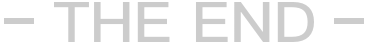```/**
* 时间美化函数
* @param [time] \$time
* @return void
*/
function format_date(\$time)
{
\$t=time()-strtotime(\$time);
\$f=array(
'31536000'=>'年',
'2592000'=>'个月',
'604800'=>'星期',
'86400'=>'天',
'3600'=>'小时',
'60'=>'分钟',
'1'=>'秒'
);

foreach (\$f as \$k=>\$v){
if (0 !=\$c=floor(\$t/(int)\$k)) {
return \$c.\$v.'前';
}
}
}```